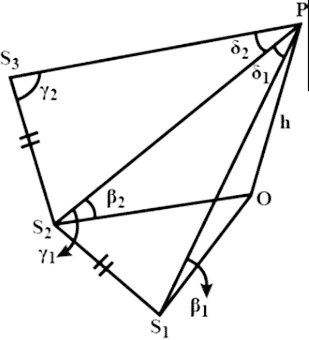A person observes the top P of a vertical tower OP of height h from a station S1 and finds that β1 is the angle of elevation. He moves in a horizontal plane to second station S2 and finds that ∠PS2S1 is γ1 and the angle subtended by S2S1 at P is δ1 and the angle of elevation is β2. He moves again to a third station S3 such that S3S2=S2S1,  ∠PS3S2=γ2 and the angle subtended by S3S2 at P is δ2. then sin⁡γ1sin⁡β1sin⁡δ1=sin⁡γ2sin⁡β2sin⁡δ2=hS1S2

# A person observes the top P of a vertical tower OP of height h from a station ${S}_{1}$ and finds that ${\beta }_{1}$ is the angle of elevation. He moves in a horizontal plane to second station S2 and finds that $\angle P{S}_{2}{S}_{1}$ is ${\gamma }_{1}$ and the angle subtended by ${S}_{2}{S}_{1}$ at P is ${\delta }_{1}$ and the angle of elevation is β2. He moves again to a third station ${S}_{3}$ such that  and the angle subtended by ${S}_{3}{S}_{2}$ at P is ${\delta }_{2}$. then $\frac{\mathit{sin}{\gamma }_{1}\mathit{sin}{\beta }_{1}}{\mathit{sin}{\delta }_{1}}=\frac{\mathit{sin}{\gamma }_{2}\mathit{sin}{\beta }_{2}}{\mathit{sin}{\delta }_{2}}=\frac{h}{{S}_{1}{S}_{2}}$

1. A
True
2. B
False

Fill Out the Form for Expert Academic Guidance!l

+91

Live ClassesBooksTest SeriesSelf Learning

Verify OTP Code (required)

### Solution:

Sine rule in given as,
$\frac{\mathit{sin}A}{a}=\frac{\mathit{sin}B}{b}=\frac{\mathit{sin}C}{c}$
Here,
a, b, c $\to$     sides of a triangle
A, B, C$\to$     Angles (lying opposite to sides a, b, c respectively.)
Use sine rule in the triangles $P{S}_{1}{S}_{2}$ and $P{S}_{2}{S}_{3}$ with the involvement of sides $P{S}_{1}{S}_{1}$ and ${S}_{2}{S}_{1}$ for triangle $P{S}_{1}{S}_{2}$ and sides $P{S}_{2}{S}_{2}$ and ${S}_{3}{S}_{2}$ for triangle $P{S}_{2}{S}_{3}$.
It is known that,
$\mathit{sin\theta }=\frac{\mathit{Perpendicular}}{\mathit{Hypotaneous}}$

From  by sine formula,
$\frac{P{S}_{1}\mathit{}}{\mathit{sin}{\gamma }_{1}\mathit{}}=\frac{{S}_{1}{S}_{2}}{\mathit{sin}{\delta }_{1}\mathit{}}$
$⇒\frac{h\mathit{}}{\mathit{sin}{\beta }_{1}\mathit{sin}{\gamma }_{1}\mathit{}}=\frac{{S}_{1}{S}_{2}}{\mathit{sin}{\delta }_{1}\mathit{}}$ ……. (1)
$⇒\frac{h\mathit{}}{{S}_{1}{S}_{2}\mathit{}}=\frac{\mathit{sin}{\beta }_{1}\mathit{sin}{\gamma }_{1}}{\mathit{sin}{\delta }_{1}\mathit{}}$In the same way,
$\frac{h\mathit{}}{{S}_{2}{S}_{3}\mathit{}}=\frac{\mathit{sin}{\beta }_{2}\mathit{sin}{\gamma }_{2}}{\mathit{sin}{\delta }_{2}\mathit{}}$
But ${S}_{2}{S}_{3}={S}_{1}{S}_{2}$   (given)
$\frac{\mathit{sin}{\beta }_{1}\mathit{sin}{\gamma }_{1}}{\mathit{sin}{\delta }_{1}\mathit{}}\mathit{}$
$=\frac{\mathit{sin}{\beta }_{2}\mathit{sin}{\gamma }_{2}}{\mathit{sin}{\delta }_{2}\mathit{}}$
$=\frac{h\mathit{}}{{S}_{1}{S}_{2}\mathit{}}$
Option 1 is Correct.

## Related content

 Area of Square Area of Isosceles Triangle Pythagoras Theorem Triangle Formula Perimeter of Triangle Formula Area Formulae Volume of Cone Formula Matrices and Determinants_mathematics Critical Points Solved Examples Type of relations_mathematics+91

Live ClassesBooksTest SeriesSelf Learning

Verify OTP Code (required)